### Home > MC2 > Chapter 2 > Lesson 2.2.3 > Problem2-69

2-69.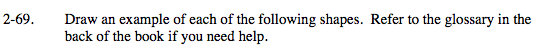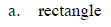What are the characteristics of a rectangle?

A rectangle has 4 sides and 4 ninety degree angles.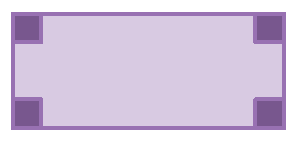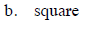What are the characteristics of a square?

A square has 4 sides of equal length and 4 ninety degree angles.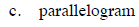What are the characteristics of a parallelogram?

A parallelogram has 4 sides with two pairs of parallel sides.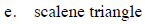What are the characteristics of a scalene triangle?

A scalene triangle has no congruent sides.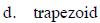What are the characteristics of a trapezoid?

A trapezoid is a quadrilateral with at least one pair of parallel sides.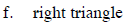What are the characteristics of a right triangle?

A right triangle has one right angle.